## Present Value of Payments

The value of a bond is obtained by discounting the bond’s expected cash flows to the present using an appropriate discount rate.

### Learning Objectives

Calculate the present value of an annuity

### Key Takeaways

#### Key Points

• The bond price can be summarized as the sum of the present value of the par value repaid at maturity and the present value of coupon payments.
• The present value of coupon payments is the present value of an annuity of coupon payments.
• The present value of an annuity is the value of a stream of payments, discounted by the interest rate to account for the payments being made at various moments in the future.

#### Key Terms

• discount rate: The interest rate used to discount future cash flows of a financial instrument; the annual interest rate used to decrease the amounts of future cash flow to yield their present value.

The bond price can be calculated using the present value approach. Bond valuation is the determination of the fair price of a bond. As with any security or capital investment, the theoretical fair value of a bond is the present value of the stream of cash flows it is expected to generate. Therefore, the value of a bond is obtained by discounting the bond’s expected cash flows to the present using an appropriate discount rate. In practice, this discount rate is often determined by reference to similar instruments, provided that such instruments exist. The formula for calculating a bond’s price uses the basic present value (PV) formula for a given discount rate.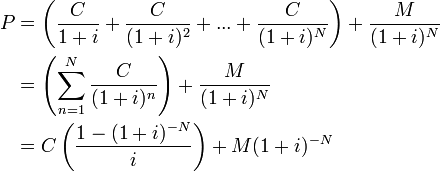Bond Price: Bond price is the present value of coupon payments and face value paid at maturity.

F = face value, iF = contractual interest rate, C = F * iF = coupon payment (periodic interest payment), N = number of payments, i = market interest rate, or required yield, or observed / appropriate yield to maturity, M = value at maturity, usually equals face value, and P = market price of bond.

The bond price can be summarized as the sum of the present value of the par value repaid at maturity and the present value of coupon payments. The present value of coupon payments is the present value of an annuity of coupon payments.

An annuity is a series of payments made at fixed intervals of time. The present value of an annuity is the value of a stream of payments, discounted by the interest rate to account for the payments being made at various moments in the future. The present value is calculated by:

${ \text{PV} }_{ \text{A} }={ \text{PMT} }_{ \text{i} }\cdot \left( 1-\frac { 1 }{ { (1+\text{i}) }^{ \text{n} } } \right)$

i is the number of periods and n is the per period interest rate.

## Par Value at Maturity

Par value is stated value or face value, with a typical bond making a repayment of par value at maturity.

### Learning Objectives

Calculate a bond’s par value

### Key Takeaways

#### Key Points

• A bond selling at par has a coupon rate such that the bond is worth an amount equivalent to its original issue value or its value upon redemption at maturity.
• A typical bond makes coupon payments at fixed intervals during the life of it and a final repayment of par value at maturity. Together with coupon payments, the par value at maturity is discounted back to the time of purchase to calculate the bond price.
• Par value of a bond usually does not change, except for inflation -linked bonds whose par value is adjusted by inflation rates every predetermined period of time.

#### Key Terms

• inflation-linked bonds: Inflation-indexed bonds (also known as inflation-linked bonds or colloquially as linkers) are bonds where the principal is indexed to inflation. They are thus designed to cut out the inflation risk of an investment.

Par value, in finance and accounting, means the stated value or face value. From this comes the expressions at par (at the par value), over par (over par value) and under par (under par value). A bond selling at par has a coupon rate such that the bond is worth an amount equivalent to its original issue value or its value upon redemption at maturity. Corporate bonds usually have par values of $1,000 while municipal bonds generally have face values of$500. Federal government bonds tend to have much higher face values at $10,000. A typical bond makes coupon payments at fixed intervals during the life of it and a final repayment of par value at maturity. Together with coupon payments, the par value at maturity is discounted back to the time of purchase to calculate the bond price. Below is the formula for calculating a bond’s price, which uses the basic present value (PV) formula for a given discount rate.Bond Price Formula: Bond price is the present value of coupon payments and the par value at maturity. F = face value, iF = contractual interest rate, C = F * iF = coupon payment (periodic interest payment), N = number of payments, i = market interest rate, or required yield, or observed/ appropriate yield to maturity, M = value at maturity, usually equals face value, P = market price of bond. Par value of a bond usually does not change, except for inflation-linked bonds whose par value is adjusted by inflation rates every predetermined period of time. The coupon payments of such bonds are also accordingly adjusted even though the coupon interest rate is unchanged. ## Yield to Maturity Yield to maturity is the discount rate at which the sum of all future cash flows from the bond are equal to the price of the bond. ### Learning Objectives Classify a bond based on its market value and Yield to Maturity ### Key Takeaways #### Key Points • The Yield to maturity is the internal rate of return earned by an investor who bought the bond today at the market price, assuming that the bond will be held until maturity, and that all coupon and principal payments will be made on schedule. • Yield to maturity (YTM) = [(Face value/Present value)1/Time period]-1. • If the YTM is less than the bond’s coupon rate, then the market value of the bond is greater than par value ( premium bond). If a bond’s coupon rate is less than its YTM, then the bond is selling at a discount. If a bond’s coupon rate is equal to its YTM, then the bond is selling at par. • There are some variants of YTM: yield to call, yield to put, yield to worst… #### Key Terms • quote: To name the current price, notably of a financial security. • internal rate of return: IRR. The rate of return on an investment which causes the net present value of all future cash flows to be zero. • call premium: the additional cost paid by the issuer for the right to buy back the bond at a predtermined price at a certain time in the future The Yield to maturity (YTM) or redemption yield of a bond or other fixed- interest security, such as gilts, is the internal rate of return (IRR, overall interest rate ) earned by an investor who buys the bond today at the market price, assuming that the bond will be held until maturity, and that all coupon and principal payments will be made on schedule.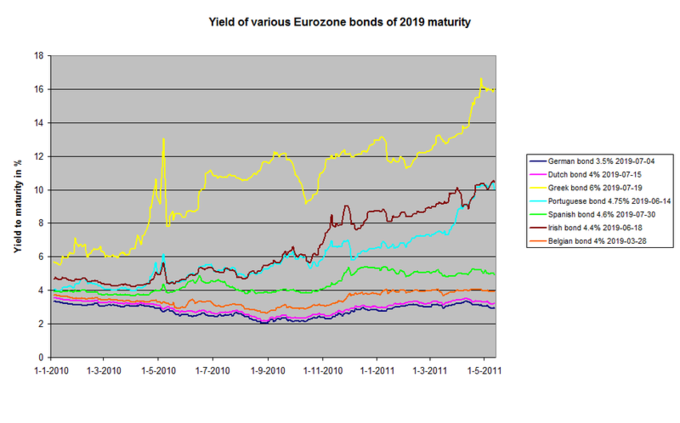Yield to Maturity: Development of yield to maturity of bonds of 2019 maturity of a number of Eurozone governments. Contrary to popular belief, including concepts often cited in advanced financial literature, Yield to maturity does not depend upon a reinvestment of dividends. Yield to maturity, rather, is simply the discount rate at which the sum of all future cash flows from the bond (coupons and principal) is equal to the price of the bond. The formula for yield to maturity: Yield to maturity (YTM) = [(Face value / Present value)1/Time period]-1 The YTM is often given in terms of Annual Percentage Rate (A.P.R.), but usually market convention is followed: in a number of major markets the convention is to quote yields semi-annually (for example, an annual effective yield of 10.25% would be quoted as 5.00%, because 1.05 x 1.05 = 1.1025). If the yield to maturity for a bond is less than the bond’s coupon rate, then the (clean) market value of the bond is greater than the par value (and vice versa). • If a bond’s coupon rate is less than its YTM, then the bond is selling at a discount. • If a bond’s coupon rate is more than its YTM, then the bond is selling at a premium. • If a bond’s coupon rate is equal to its YTM, then the bond is selling at par. As some bonds have different characteristics, there are some variants of YTM: • Yield to call: when a bond is callable (can be repurchased by the issuer before the maturity), the market looks also to the Yield to call, which is the same calculation of the YTM, but assumes that the bond will be called, so the cash flow is shortened. • Yield to put: same as yield to call, but when the bond holder has the option to sell the bond back to the issuer at a fixed price on specified date. • Yield to worst: when a bond is callable, puttable, exchangeable, or has other features, the yield to worst is the lowest yield of yield to maturity, yield to call, yield to put, and others. For instance, you buy ABC Company bond which matures in 1 year and has a 5% interest rate (coupon) and has a par value of$100. You pay $90 for the bond. The current yield is 5.56% ((5/90)*100). If you hold the bond until maturity, ABC Company will pay you$5 as interest and $100 par value for the matured bond. Now for your$90 investment, you get $105, so your yield to maturity is 16.67% [= (105/90)-1] or [=(105-90)/90]. ## Inflation Premium An inflation premium is the part of prevailing interest rates that results from lenders compensating for expected inflation. ### Learning Objectives Explain how to determine and use an inflation premium ### Key Takeaways #### Key Points • Investors seek this premium to compensate for the erosion in the value of their capital due to inflation. • Actual interest rates (without factoring in inflation) are viewed by economists and investors as being the nominal (stated) interest rate minus the inflation premium. • Letting r denote the real interest rate, i denote the nominal interest rate, and let π denote the inflation rate, the Fisher equation is: i = r + π. In the Fisher equation, π is the inflation premium. #### Key Terms • systematic risks: In finance and economics, systematic risk (sometimes called aggregate risk, market risk, or undiversifiable risk) is vulnerability to events which affect aggregate outcomes such as broad market returns, total economy-wide resource holdings, or aggregate income. An inflation premium is the part of prevailing interest rates that results from lenders compensating for expected inflation by pushing nominal interest rates to higher levels.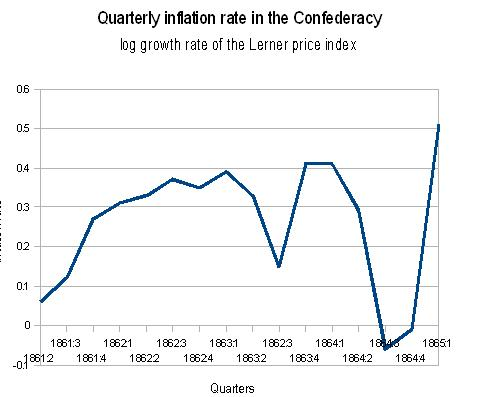Inflation rate graph: Inflation rate in the Confederacy during the American Civil War. In economics and finance, an individual who lends money for repayment at a later point in time expects to be compensated for the time value of money, or not having the use of that money while it is lent. In addition, they will want to be compensated for the risks of the money having less purchasing power when the loan is repaid. These risks are systematic risks, regulatory risks and inflationary risks. The first includes the possibility that the borrower will default or be unable to pay on the originally agreed upon terms, or that collateral backing the loan will prove to be less valuable than estimated. The second includes taxation and changes in the law which would prevent the lender from collecting on a loan or having to pay more in taxes on the amount repaid than originally estimated. The third takes into account that the money repaid may not have as much buying power from the perspective of the lender as the money originally lent, that is inflation, and may include fluctuations in the value of the currencies involved. The inflation premium will compensate for the third risk, so investors seek this premium to compensate for the erosion in the value of their capital, due to inflation. Actual interest rates (without factoring in inflation) are viewed by economists and investors as being the nominal (stated) interest rate minus the inflation premium. The Fisher equation in financial mathematics and economics estimates the relationship between nominal and real interest rates under inflation. In economics, this equation is used to predict nominal and real interest rate behavior. Letting r denote the real interest rate, i denote the nominal interest rate, and let π denote the inflation rate, the Fisher equation is: i = r + π. In the Fisher equation, π is the inflation premium. For example, if an investor were able to lock in a 5% interest rate for the coming year and anticipates a 2% rise in prices, he would expect to earn a real interest rate of 3%. 2% is the inflation premium. This is not a single number, as different investors have different expectations of future inflation. Since the inflation rate over the course of a loan is not known initially, volatility in inflation represents a risk to both the lender and the borrower. ## Differences Between Real and Nominal Rates Nominal rate refers to the rate before adjustment for inflation; the real rate is the nominal rate minus inflation: r = R – i or, 1+r = (1+r)(1+E(r)). ### Learning Objectives Differentiate between real and nominal interest rates ### Key Takeaways #### Key Points • Nominal rate refers to the rate before adjustment for inflation; the real rate is the nominal rate minus inflation. • Fisher equation states that the real interest rate is approximately the nominal interest rate minus the inflation rate: 1 + i = (1+r) (1+E(r)). • Simple equation between nominal rates and real rates: i = R – r. #### Key Terms • purchasing power: Purchasing power (sometimes retroactively called adjusted for inflation) is the amount of goods or services that can be purchased with a unit of currency. In finance and economics, nominal rate refers to the rate before adjustment for inflation (in contrast with the real rate). The real rate is the nominal rate minus inflation. In the case of a loan, it is this real interest that the lender receives as income. If the lender is receiving 8% from a loan and inflation is 8%, then the real rate of interest is zero, because nominal interest and inflation are equal. A lender would have no net benefit from such a loan because inflation fully diminishes the value of the loan’s profit. The relationship between real and nominal rates can be described in the equation: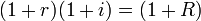Real and nominal: The relationship between real and nominal interest rates is captured by the formula. Where r is the real rate, i is the inflation rate, and R is the nominal rate. The real rate can be described more formally by the Fisher equation, which states that the real interest rate is approximately the nominal interest rate minus the inflation rate: 1 + i = (1+r) (1+E(r)), where i = nominal interest rate; r = real interest rate; E(r) = expected inflation rate. For example, if somebody lends$1,000 for a year at 10%, and receives $1,100 back at the end of the year, this represents a 10% increase in his purchasing power if prices for the average goods and services that he buys are unchanged from what they were at the beginning of the year. However, if the prices of the food, clothing, housing, and other things that he wishes to purchase have increased 20% over this period, he has in fact suffered a real loss of about 12% in his purchasing power. In this analysis, the nominal rate is the stated rate, and the real rate is the rate after the expected losses due to inflation. Since the future inflation rate can only be estimated, the ex ante and ex post (before and after the fact) real rates may be different; the premium paid to actual inflation may be higher or lower. ## Time to Maturity “Time to maturity” refers to the length of time before the par value of a bond must be returned to the bondholder. ### Learning Objectives Discuss the importance of a bond’s maturity when determining its value ### Key Takeaways #### Key Points • The maturity can be any length of time, but debt securities with a term of less than one year are generally not designated as bonds. Instead, they are considered money market instruments. • In the market for United States Treasury securities, there are three categories of bond maturities: short-term, medium-term and long-term bonds. • A bond that takes longer to mature necessarily has a greater duration. The bond price in this type of a situation, therefore, is more sensitive to changes in interest rates. #### Key Terms • duration: A measure of the sensitivity of the price of a financial asset to changes in interest rates, computed for a simple bond as a weighted average of the maturities of the interest and principal payments associated with it • money market: A market for trading short-term debt instruments, such as treasury bills, commercial paper, bankers’ acceptances, and certificates of deposit ### Time to Maturity “Time to maturity” refers to the length of time that can elapse before the par value (face value) for a bond must be returned to a bondholder. This time may be as short as a few months, or longer than 50 years. Once this time has been reached, the bondholder should receive the par value for their particular bond. The issuer of a bond has to repay the nominal amount for that bond on the maturity date. After this date, as long as all due payments have been made, the issuer will have no further obligations to the bondholders. The length of time until a bond’s matures is referred to as its term, tenor, or maturity. These dates can technically be any length of time, but debt securities with a term of less than one year are generally not designated as bonds. Instead, they are designated as money market instruments.Money market interest rates: Interest rates of one-month maturity of German banks from 1967 to 2003 Most bonds have a term of up to 30 years. That being said, bonds have been issued with terms of 50 years or more, and historically, issues have arisen where bonds completely lack maturity dates (irredeemables). In the market for United States Treasury securities, there are three categories of bond maturities: • Short term (bills): maturities between one to five years (Instruments that mature in less than one year are considered Money Market Instruments. ) • Medium term (notes): maturities between six to twelve years • Long term (bonds): maturities greater than twelve years Because bonds with long maturities necessarily have long durations, the bond prices in these situations are more sensitive to interest rate changes. In other words, the price risk of such bonds is higher. The fair price of a “straight bond,” a bond with no embedded options, is usually determined by discounting its expected cash flows at the appropriate discount rate. Although this present value relationship reflects the theoretical approach to determining the value of a bond, in practice, the price is (usually) determined with reference to other, more liquid instruments. In general, coupon and par value being equal, a bond with a short time to maturity will trade at a higher value than one with a longer time to maturity. This is because the par value is discounted at a higher rate further into the future. Finally, it is important to recognize that future interest rates are uncertain, and that the discount rate is not adequately represented by a single fixed number (this would be the case if an option was written on the bond in question) stochastic calculus may be employed. Where the market price of a bond is less than its face value (par value), the bond is selling at a discount. Conversely, if the market price of bond is greater than its face value, the bond is selling at a premium. ## Calculating Yield to Maturity Using the Bond Price The yield to maturity is the discount rate that returns the bond’s market price: YTM = [(Face value/Bond price)1/Time period]-1. ### Learning Objectives Calculate a bond’s yield to maturity ### Key Takeaways #### Key Points • To achieve a return equal to YTM (i.e., where it is the required return on the bond ), the bond owner must buy the bond at price P0, hold the bond until maturity, and redeem the bond at par. • If a bond’s coupon rate is less than its YTM, then the bond is selling at a discount. If a bond’s coupon rate is more than its YTM, then the bond is selling at a premium. If a bond’s coupon rate is equal to its YTM, then the bond is selling at par. • Formula for yield to maturity: Yield to maturity(YTM) = [(Face value/Bond price)1/Time period ]-1. #### Key Terms • discount rate: The interest rate used to discount future cash flows of a financial instrument; the annual interest rate used to decrease the amounts of future cash flow to yield their present value. ### YTM The yield to maturity is the discount rate which returns the market price of the bond. YTM is the internal rate of return of an investment in the bond made at the observed price.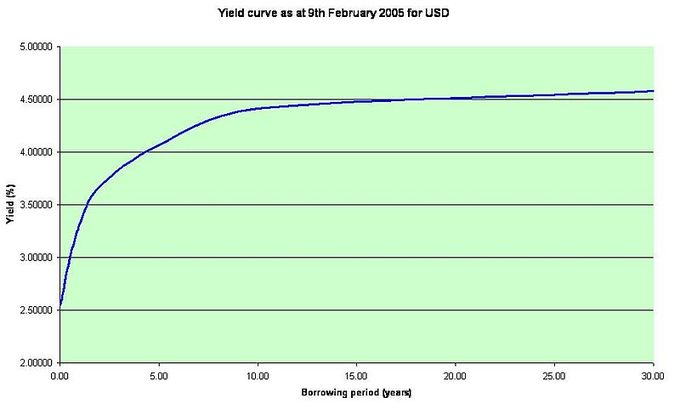USD Yield Curve: 2005 USD yield curve To achieve a return equal to YTM (i.e., where it is the required return on the bond), the bond owner must buy the bond at price P0, hold the bond until maturity, and redeem the bond at par. If the yield to maturity for a bond is less than the bond’s coupon rate, then the (clean) market value of the bond is greater than the par value (and vice versa). If a bond’s coupon rate is less than its YTM, then the bond is selling at a discount. If a bond’s coupon rate is more than its YTM, then the bond is selling at a premium. If a bond’s coupon rate is equal to its YTM, then the bond is selling at par. ### Calculating YTM Formula for yield to maturity: Yield to maturity(YTM) = [(Face value/Bond price)1/Time period]-1 As can be seen from the formula, the yield to maturity and bond price are inversely correlated. Consider a 30-year, zero-coupon bond with a face value of$100. If the bond is priced at an annual YTM of 10%, it will cost $5.73 today (the present value of this cash flow, 100/(1.1)30 = 5.73). Over the coming 30 years, the price will advance to$100, and the annualized return will be 10%.

What happens in the meantime? Suppose that over the first 10 years of the holding period, interest rates decline, and the yield-to-maturity on the bond falls to 7%. With 20 years remaining to maturity, the price of the bond will be 100/1.0720, or $25.84. Even though the yield-to-maturity for the remaining life of the bond is just 7%, and the yield-to-maturity bargained for when the bond was purchased was only 10%, the return earned over the first 10 years is 16.25%. This can be found by evaluating (1+i) from the equation (1+i)10 = (25.842/5.731), giving 1.1625. Over the remaining 20 years of the bond, the annual rate earned is not 16.25%, but rather 7%. This can be found by evaluating (1+i) from the equation (1+i)20 = 100/25.84, giving 1.07. Over the entire 30 year holding period, the original$5.73 invested increased to \$100, so 10% per annum was earned, irrespective of any interest rate changes in between.

## Impact of Payment Frequency on Bond Prices

Payment frequency can be annual, semi annual, quarterly, or monthly; the more frequently a bond makes coupon payments, the higher the bond price.

### Learning Objectives

Calculate the price of a bond

### Key Takeaways

#### Key Points

• Payment frequency can be annual, semi annual, quarterly, monthly, weekly, daily, or continuous.
• Bond price is the sum of the present value of face value paid back at maturity and the present value of an annuity of coupon payments. The present value of face value received at maturity is the same. However, the present values of annuities of coupon payments vary among payment frequencies.
• The more frequent a bond makes coupon payments, the higher the bond price, given equal coupon, par, and face.

#### Key Terms

• annuity: A specified income payable at stated intervals for a fixed or a contingent period, often for the recipient’s life, in consideration of a stipulated premium paid either in prior installment payments or in a single payment. For example, a retirement annuity paid to a public officer following his or her retirement.

The payment schedule of financial instruments defines the dates at which payments are made by one party to another on, for example, a bond or a derivative. It can be either customised or parameterized. Payment frequency can be annual, semi annual, quarterly, monthly, weekly, daily, or continuous.

Bond prices is the present value of all coupon payments and the face value paid at maturity. The formula to calculate bond prices:Bond price formula: Bond price is the present value of all coupon payments and the face value paid at maturity.

F = face value, iF = contractual interest rate, C = F * iF = coupon payment (periodic interest payment), N = number of payments, i = market interest rate, or required yield, or observed / appropriate yield to maturity, M = value at maturity, usually equals face value, P = market price of bond.

In other words, bond price is the sum of the present value of face value paid back at maturity and the present value of an annuity of coupon payments. For bonds of different payment frequencies, the present value of face value received at maturity is the same. However, the present values of annuities of coupon payments vary among payment frequencies.

The present value of an annuity is the value of a stream of payments, discounted by the interest rate to account for the payments are being made at various moments in the future. The formula is: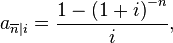Annuity formula: The formula to calculate PV of annuities.

Where n is the number of terms or number of payments n =1 (annually), n = 2 (semi-annually), n = 4 (quarterly)… and i is the per period interest rate.

According to the formula, the greater n, the greater the present value of the annuity (coupon payments). To put it differently, the more frequent a bond makes coupon payments, the higher the bond price.

## Deciding to Refund Bonds

Refunding occurs when an entity that has issued callable bonds calls those debt securities to issue new debt at a lower coupon rate.

### Learning Objectives

Explain when to refund a debt issue

### Key Takeaways

#### Key Points

• The issue of new, lower- interest debt allows the company to prematurely refund the older, higher-interest debt.
• Bond refunding occurs when a) interest rates in the market are sufficiently less than the coupon rate on the old bond, b) the price of the old bond is less than par. and c) the sinking fund has accumulated enough money to retire the bond issue.
• The decision of whether to refund a particular debt issue is usually based on a capital budgeting ( present value ) analysis.

#### Key Terms

• sinking fund: A sinking fund is a fund established by a government agency or business for the purpose of reducing debt by repaying or purchasing outstanding loans and securities held against the entity. It helps keep the borrower liquid so it can repay the bondholder.

Refunding occurs when an entity that has issued callable bonds calls those debt securities from the debt holders with the express purpose of reissuing new debt at a lower coupon rate. In essence, the issue of new, lower-interest debt allows the company to prematurely refund the older, higher-interest debt. On the contrary, nonrefundable bonds may be callable, but they cannot be re-issued with a lower coupon rate (i.e., they cannot be refunded).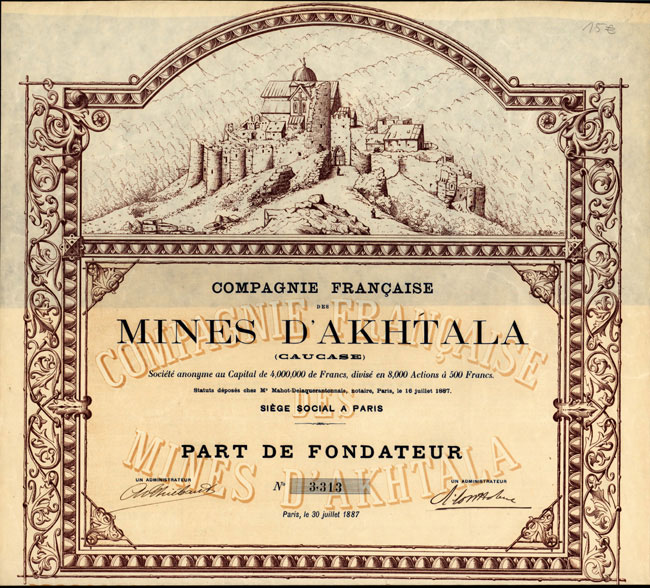French Bond: French Bond for the Akhtala mines issued in 1887.

The decision of whether to refund a particular debt issue is usually based on a capital budgeting (present value) analysis. The principal benefit, or cash inflow, is the present value of the after-tax interest savings over the life of the issue.

Bond refunding occurs when all three of the following are true

1. Interest rates in the market are sufficiently less than the coupon rate on the old bond
2. The price of the old bond is less than par
3. The sinking fund has accumulated enough money to retire the bond issue.

The three steps of whether to make a refunding decision are as follows:

Step 1: Calculate the present value of interest savings (cash inflows):

Interest savings = annual interest of old issue – annual interest of new issue

Step 2: Calculate the net investment (net cash outflow at time 0). This involves computing the after-tax call premium, the issuance cost of the new issue, the issuance cost of the old issue, and the overlapping interest. The call premium is a cash outflow.

Step 3: Finally, calculate the net present value of refunding.

Net present value of refunding = Present value of interest savings – Present value of net investment